Home > AC > Chapter 11 > Lesson 11.1.1 > Problem11-9

11-9.
1. Find the slope (m) and y-intercept (b) for each line below. Homework Help ✎

1. 2x + 7y = 14

2.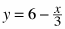3.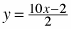4. y = 3x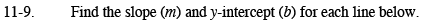Rewrite each equation in Slope-Intercept, or y = mx + b, form.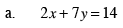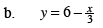$\text{This is equivalent to } y= -\frac{1}{3}x+6$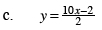$\text{This is equivalent to }y=\frac{10}{2}x-\frac{2}{2}.$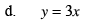This is equivalent to y = 3x + 0